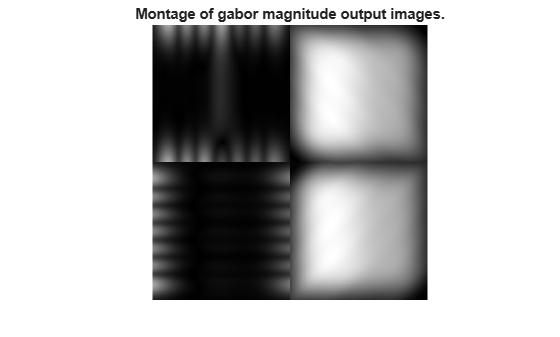# gabor

Create Gabor filter or Gabor filter bank

## Description

A gabor object represents a linear Gabor filter that is sensitive to textures with a specified wavelength and orientation.

You can use the gabor function to create a single Gabor filter or a Gabor filter bank. A filter bank is a set of filters that represent combinations of multiple wavelengths, orientations, and other optional parameters. For example, if you specify two wavelengths and three orientations, then the Gabor filter bank consists of six filters for each combination of wavelength and orientation.

To apply a Gabor filter or a Gabor filter bank to an image, use the imgaborfilt function.

## Creation

### Syntax

g = gabor(Wavelength,Orientation)
g = gabor(wavelength,orientation,Name,Value)

### Description

example

g = gabor(Wavelength,Orientation) creates a Gabor filter and sets the Wavelength and Orientation properties with the wavelength and orientation of the filter.If you specify Wavelength or Orientation as vectors, then the gabor function creates an array of gabor objects that contain all the unique combinations of Wavelength and Orientation.
g = gabor(wavelength,orientation,Name,Value) also uses name-value pairs to set one or both of the SpatialFrequencyBandwidth and SpatialAspectRatio properties. You can specify multiple name-value pairs. Enclose each property name in quotes.If you specify SpatialFrequencyBandwidth or SpatialAspectRatio as vectors, then the gabor function creates an array of gabor objects that represent all combinations of the input argument values.Example: gabor(wavelength,orientation,'SpatialFrequencyBandwidth',2) creates a Gabor filter with a spatial frequency bandwidth of two octaves.

## Properties

expand all

Wavelength of the sinusoidal carrier, specified as a numeric scalar or numeric vector with values greater than or equal to 2, in pixels/cycle. Typical values of Wavelength range from 2 up to the hypotenuse length of the input image .

You cannot change the Wavelength property after creating the gabor object.

Orientation of filter in degrees, specified as a numeric scalar or numeric vector with values in the range [0, 360]. The orientation is defined as the normal direction to the sinusoidal plane wave.

If you are interested in only the Gabor magnitude response, then restrict the range of Orientation to [0, 180].

You cannot change the Orientation property after creating the gabor object.

Spatial-frequency bandwidth in units of octaves, specified as a positive number or a vector of positive numbers. The spatial-frequency bandwidth determines the cutoff of the filter response as frequency content in the input image varies from the preferred frequency, 1/lambda. Typical values for the spatial-frequency bandwidth are in the range [0.5, 2.5].

You cannot change the SpatialFrequencyBandwidth property after creating the gabor object.

Ratio of the semimajor and semiminor axes of the Gaussian envelope (semiminor/semimajor), specified as a positive number or a vector of positive numbers. This property controls the ellipticity of the Gaussian envelope. Typical values for spatial aspect ratio are in the range [0.23, 0.92].

You cannot change the SpatialAspectRatio property after creating the gabor object.

Spatial kernel, specified as a numeric matrix.

## Examples

collapse all

Create a sample image of a checkerboard.

A = checkerboard(20);

Create an array of Gabor filters.

wavelength = 20; orientation = [0 45 90 135]; g = gabor(wavelength,orientation);

Apply the filters to the checkerboard image.

outMag = imgaborfilt(A,g);

Display the results.

outSize = size(outMag); outMag = reshape(outMag,[outSize(1:2),1,outSize(3)]); figure, montage(outMag,'DisplayRange',[]); title('Montage of gabor magnitude output images.');Create array of Gabor filters.

g = gabor([5 10],[0 90]);

Visualize the real part of the spatial convolution kernel of each Gabor filter in the array.

figure; subplot(2,2,1) for p = 1:length(g) subplot(2,2,p); imshow(real(g(p).SpatialKernel),[]); lambda = g(p).Wavelength; theta = g(p).Orientation; title(sprintf('Re[h(x,y)], \\lambda = %d, \\theta = %d',lambda,theta)); endJain, Anil K., and Farshid Farrokhnia. "Unsupervised Texture Segmentation Using Gabor Filters." Pattern Recognition 24, no. 12 (January 1991): 1167–86. https://doi.org/10.1016/0031-3203(91)90143-S.